MathScore EduFighter is one of the best math games on the Internet today. You can start playing for free!

## Triangle Area - Sample Math Practice Problems

The math problems below can be generated by MathScore.com, a math practice program for schools and individual families. References to complexity and mode refer to the overall difficulty of the problems as they appear in the main program. In the main program, all problems are automatically graded and the difficulty adapts dynamically based on performance. Answers to these sample questions appear at the bottom of the page. This page does not grade your responses.

See some of our other supported math practice problems.

### Complexity=1

Find the surface area and volume. Answer with proper units. To represent m2, use "sq m". Sample answer: "5 sq m". To represent m3, use "cu m".

 1.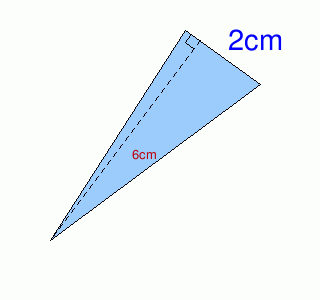area = 2.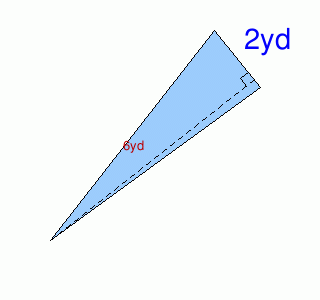area =

### Complexity=2

Find the surface area and volume. Answer with proper units. To represent m2, use "sq m". Sample answer: "5 sq m". To represent m3, use "cu m".

 1.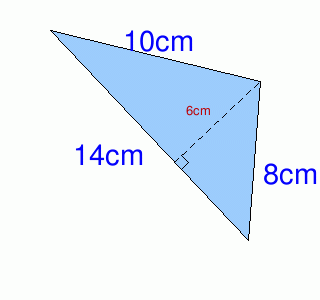area = 2.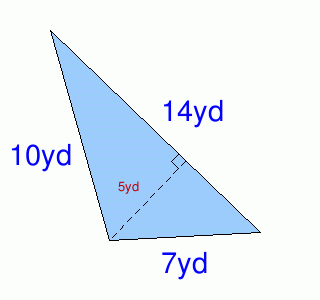area =

### Complexity=3

Find the surface area and volume. Answer with proper units. To represent m2, use "sq m". Sample answer: "5 sq m". To represent m3, use "cu m".

 1.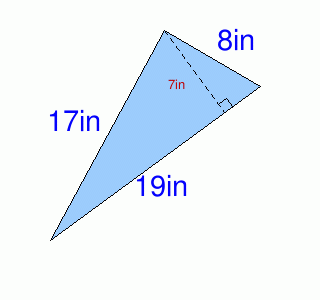area = 2.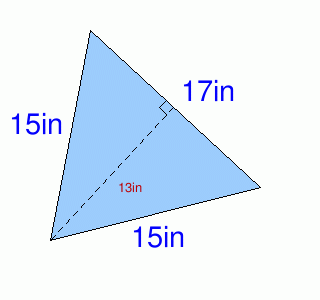area =

### Complexity=4

Find the surface area and volume. Answer with proper units. To represent m2, use "sq m". Sample answer: "5 sq m". To represent m3, use "cu m".

 1.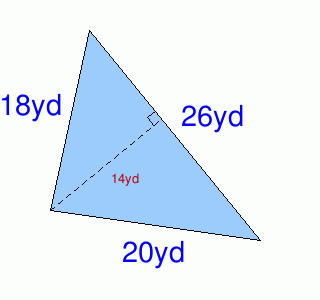area = 2.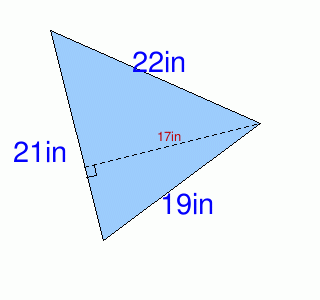area =

### Complexity=1

Find the surface area and volume. Answer with proper units. To represent m2, use "sq m". Sample answer: "5 sq m". To represent m3, use "cu m".

1area =
Solution
A=1/2bh (one half base times height)
= 1/2 * 2cm * 6cm = 6 cm2.
2area =
Solution
A=1/2bh (one half base times height)
= 1/2 * 2yd * 6yd = 6 yd2.

### Complexity=2

Find the surface area and volume. Answer with proper units. To represent m2, use "sq m". Sample answer: "5 sq m". To represent m3, use "cu m".

1area =
Solution
A=1/2bh (one half base times height)
= 1/2 * 14cm * 6cm = 42 cm2.
2area =
Solution
A=1/2bh (one half base times height)
= 1/2 * 14yd * 5yd = 35 yd2.

### Complexity=3

Find the surface area and volume. Answer with proper units. To represent m2, use "sq m". Sample answer: "5 sq m". To represent m3, use "cu m".

1area =
Solution
A=1/2bh (one half base times height)
= 1/2 * 19in * 7in = 66.5 in2.
2area =
Solution
A=1/2bh (one half base times height)
= 1/2 * 17in * 13in = 110.5 in2.

### Complexity=4

Find the surface area and volume. Answer with proper units. To represent m2, use "sq m". Sample answer: "5 sq m". To represent m3, use "cu m".

1area =
Solution
A=1/2bh (one half base times height)
= 1/2 * 26yd * 14yd = 182 yd2.
2area =